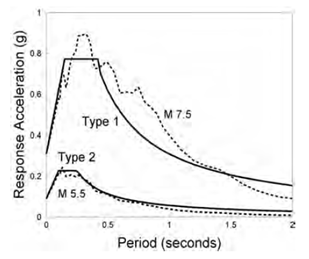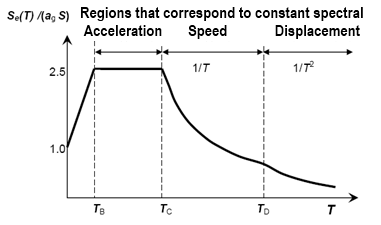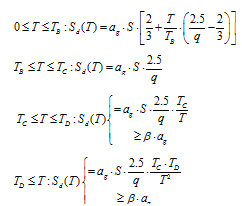US: Tel: 1-206-279-3300
EU: Tel: +30 6986 007 252
• FREE VERSION OF RCsolver - Concrete Design with EC2, EC8, and ACI 318

Get full capabiltiies with our free version of RC-Solver once you signup for our newsletter. With the free version you will have to wait 30 seconds for advertisements.

# Design spectrum according to Eurocode 8

Design specrum in the horizontal direction

The horizontal design forces are defined in Eurocode 8 from the maximum response acceleration of the structure, under the expected earthquake, that is represented with the acceleration spectrum of the structure. The starting point is an elastic response spectrum, which is reduced with factors that take into consideration the ability of the structure to absorb seismic energy through rigid deformations. The design acceleration spectrum comes from the elasticity spectrum with a depreciation of 5%, by dividing the spectral accelerations by the behavior factor q. In the horizontal plane, the seismic action acts simultaneously and independently in two orthogonal directions that have the same response spectrum.

Eurocode 8 suggests two different design spectrums, Type 1 for the more seismically active  regions of southern Europe, and Type 2 for the less seismic regions of central and northern Europe. Spectrum type 1 refers to earthquake sizes close to M7 while  spectrum type 2 is suitable for earthquakes up size M5.5.

Figure 1 presents average spectral ordinate values from the equations of seismic motion prediction of the European territory by Ambraseys et al. (1996) for rock locations distanced 10 km from small and middle sized earthquakes, in comparison with the spectrum for rock type 1 and type 2 of Eurocode 8, based on the average prediction values of the maximum soil acceleration (PGA).Figure 1: Average spectral ordinate values by Ambraseys in comparison with Eurocode 8(Elghazouli, 2009)

The elastic acceleration spectrum with a damping of 5% of Eurocode 8is given graphically below. It contains an area of fixed spectral acceleration, between the periods ΤΒ and ΤC with a value 2.5 times the maximum soil acceleration agS, that is followed from an area of fixed spectral velocity between the periods ΤC and ΤD, where the spectral acceleration is proportional to 1/Τ, and an area of fixed spectral displacement, where the spectral acceleration is proportional to 1/Τ2.Figure 2: Elastic spectrum EC in the horizontal direction for a damping of 5% (Fardis, 2009a)

In the areas of fixed spectral acceleration, velocity, and displacement, the design spectrum originates from an elastic response with a 5% damping divided by q. Exceptionally, the increasing part for a vibration period from Τ up to Τ≤ΤΒ comes from the linear interpolation between: (α) the maximum ground acceleration Sag, divided by 1.5, that expresses overstrength compared with the design capacity and the fixed design acceleration, for Τ=0 and (β) 2.5 ag/q for Τ=ΤΒ. Moreover, there is a lower limit in the design spectral acceleration, equal to the 20% of the maximum acceleration on the rock, ag. (Fardis, 2009a)

The following equations describe the design acceleration spectrum (EC8 § 3.2.2.5(4)) :where:

Sd (T)  is the design spectrum

T  is the vibration period of a linear single-degree-of-freedom system

ag is the design ground acceleration on type Α ground (agI∙agR)

γI is the importance factor of the building

TB is the lower limit of the period of the constant spectral acceleration branch

TC is the upper limit of the period of the constant spectral acceleration branch

TD is the value defining the beginning of the constant displacement response range

of the spectrum

S   is the soil factor

η  is the damping correction factor with a reference value of η = 1 for 5% viscous

damping

q  is the behaviour factor

β  is the lower limit of the seismic acceleration (suggested value β=0.2)

Design spectrum in the vertical direction

The importance of the vertical seismic component in designing structures is open to discussion, but there are certain types of structures or structural members like cantilever beams, for which the vertical action could be critical. Many earthquake standards do not have reference to the vertical elastic or design spectrum and the ones that have, they present it as the horizontal spectrum multiplied by a reduction factor (usually 1/3). Measures of seismic accelerations near the fault have shown that short term the vertical component of the seismic action can be greater than the horizontal one. In addition, it is generally acceptable that frequency content of the vertical response spectrum is different than the horizontal one (Bozorgnia and Campbell, 2004). So, Eurocode 8(§3.2.2.3) has the advantage that it defines the vertical response spectrum independently and not depending on the horizontal spectrum.

The design spectrum of the vertical seismic component is given from the same equations that define the horizontal component, with the difference that the ground factor S is considered equal to 1.0, the behavior factor q is allowed to be considered greater than 1.5 (except from the case that it is documented by appropriate study), the values of the periods TB, TC, TD are different (they are presented in the Table below), the maximum vertical acceleration avg replaces the ag, in the way that is presented in the same Table, and all the other parameters are received as presented in the previous paragraph.

 Spectrum Type avg/ ag TB TC TD Ι 0.90 0.05 0.15 1.0 ΙΙ 0.45 0.05 0.15 1.0

Table 1: Parameters of the vertical elastic response spectrum according to

EC8 (EC8 § 3.2.2.3 Table 3.4)

# E-mail List Signup

Signup to our Email List for the latest information about our products, support and more.